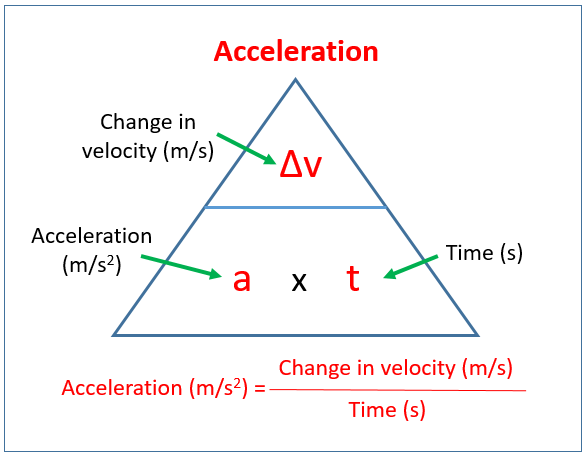# Science: Speed, Velocity and Acceleration

• Average Speed: distance travelled per unit of time (m/s)
• Velocity: displacement per unit of time (m/s)
• Average Speed is SCALAR, while velocity is a VECTOR

## Distance-Speed-Time Triangles• Usage:
• Average Speed $$=$$ Distance $$\div$$ Time
• Distance $$=$$ Average Speed $$\times$$ Time
• Time $$=$$ Distance $$\div$$ Average Speed

## Acceleration

• Acceleration is the rate at which an object’s velocity CHANGES
• Acceleration is measured in $$m/s^2$$ (metres per second squared)
• Acceleration can be found by dividing the applied force by the mass of the object
• $$a = F/m$$• Acceleration is a VECTOR quantity.
Mastodon